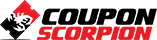# Excel Formulas And Functions With Excel Formulas Cheat Sheet

Learn Excel formulas and functions from scratch. Download Excel formulas and functions cheat sheet for your daily use. – Free Course

### What you’ll learn

• Build the foundations of Excel Formulas and Functions from scratch
• Use the Subtotal function effectively
• Work with Relative, Absolute and Mixed cell references
• Use formulas with Excel Tables
• Handle Circular References with Excel
• Use DATEDIF() and Date related Excel functions with ease

### Requirements

• Microsoft Excel 2013 or Office 2013 installed in the computer
• Students should have experience with Excel environment

### Description

Teaching 11 Courses on Excel and Data Analysis!

OVER 25,000 visitors visit my blog ExcelDemy dot com every month!!

OVER 15,000 successful students have already taken my online courses since November, 2015 with 375 total Reviews!!!

Course Updated:  Nov 01. 2016

Formulas and worksheet functions are the heart of Microsoft Excel spreadsheet. They are essential to manipulating and obtaining useful information and insight from your data.

Without formulas and functions, Excel spreadsheet is simply a fancy word-processing document. Welcome to my Excel Formulas and Functions for Beginners course!

I designed this course for beginner level Excel users. But are you an intermediate level Excel user? Want to have a broader, deeper and in-depth knowledge in building Excel formulas and functions? This course is also for you!

I am Kawser Ahmed, your instructor with this course. I am a full time Excel, Excel VBA and Data Analytics blogger. And I am a corporate Excel trainer in my own country. Monthly more than 15,000 Excel users visit my Excel blog ExcelDemy and read my Excel and data related articles and tutorials.

Lets discuss now what you will learn from this course.

1. Operators and Precedence of Operators: I will start from zero and if you dont know about the operators and precedence of operators that Excel uses in its formulas, then it will be tough for you to decode complex Excel formulas. So I have covered them very details.
2. How to use built-in Excel functions: Then you will learn how to use built-in excel functions into the formulas using Insert Function and Function Arguments dialog box.
3. Subtotal Function: You will find many uses of Subtotal function if you are using Excel with your Finance related jobs. So this function is covered in details at the start of the course.
4. Relative, Absolute and Mixed Cell References: You will work with these three concepts time to time when you will build excel formulas. So, they have got a good time in the course.
5. Formulas with Tables: Understanding how formulas work with Excel tables is very important. I have used two lectures to cover formulas with tables. In the second lecture, structured referencing with Excel tables has been discussed.
6. Circular Referencing: Then you will learn how to handle circular references with Excel formulas, how named cells and ranges work with formulas, and so on.
7. DATEDIF() and Date related Excel Functions: And I will finish this course with DATEDIF () and other Date related Excel functions!

I am assuring you that what you will learn in this crash course is priceless. I designed this course keeping in mind, to give you a solid base in Excel formulas and function. Thanks for watching. Enjoy the course!

Author(s): Kawser Ahmed

Deal Score+6
FREE 0